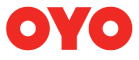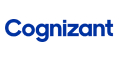New update is available. Click here to update.
Topics

# Implementation: HashMap

Easy0/40
Average time to solve is 30m+27 more companies

## Problem statement

Design a data structure that stores a mapping of a key to a given value and supports the following operations in constant time.

``````1. INSERT(key, value): Inserts an integer value to the data structure against a string type key if not already present. If already present, it updates the value of the key with the new one. This function will not return anything.

2. DELETE(key): Removes the key from the data structure if present. It doesn't return anything.

3. SEARCH(key): It searches for the key in the data structure. In case it is present, return true. Otherwise, return false.

4. GET(key): It returns the integer value stored against the given key. If the key is not present, return -1.

5. GET_SIZE(): It returns an integer value denoting the size of the data structure.

6. IS_EMPTY(): It returns a boolean value, denoting whether the data structure is empty or not.
``````
Note :
``````1. Key is always a string value.
2. Value can never be -1.
``````
Operations Performed :
``````First(Denoted by integer value 1):  Insertion to the Data Structure. It is done in a pair of (key, value).

Second(Denoted by integer value 2):  Deletion of a key from the Data Structure.

Third(Denoted by integer value 3): Search a given key in the Data Structure.

Fourth(Denoted by integer value 4): Retrieve the value for a given key from the Data Structure.

Fifth(Denoted by integer value 5): Retrieve the size of the Data Structure.

Sixth(Denoted by integer value 6): Retrieve whether the Data Structure is empty or not.
``````
Detailed explanation ( Input/output format, Notes, Images )
Constraints :
``````1 <= N <= 10 ^ 5
1 <= T <= 3
1 <= V <= 10 ^ 5

Where 'T' is the type of operation and 'V' is the value of the operand.

Time Limit: 3 sec
``````
Sample Input 1 :
``````3
1 qwerty 35
1 qwerty 50
5
``````
Sample Output 1 :
``````1
``````
Explanation Of Sample Input 1 :
``````1 operation: We need to insert 'qwerty' with a value of 35.

2 operation: We need to insert 'qwerty' with a value of 50.

3 operation: We need to return the size of HashMap. So, We will return 1.
``````
Sample Input 2 :
``````6
1 code 9
3 code
2 code
3 code
5
6
``````
Sample Output 2 :
``````true
false
0
true
``````Console